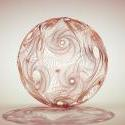1.## pxptpw

Members

4

• ### Content count

48

2.## Thomas Helzle

Members

3

• ### Content count

248

3.## Atom

Members

2

• ### Content count

3,244

4.Members

2

758

## Popular Content

Showing most liked content on 12/03/2018 in all areas

1. 4 points
anyone interested in the h17 pyro custom velocity.. normal_tools.hiplc
2. 2 points
"Folded Paper Flow" Before heading for London (see above post) I went to a local art shop and found a book about the paper-art of Richard Sweeney. I was totally fascinated by the flow and forms he creates just from folded paper. Today I finally got to do some initial experiments with similar approaches in Houdini: Created in Houdini 17, rendered in Redshift (Brute Force GI this time) and post in Luminar 2018. Cheers, Tom
3. 2 points
if you go to Edit > preferences > hip file options, you can check if your units are set to 1 meter and 1 kg. (this is standard for houdini). Then IF you modelled to real world scale you will get accurate values in meters as described above by LaidlawFX
4. 1 point
5. 1 point
"Weaving" Created in SideFX Houdini 16.5, rendered with Redshift and post done in Luminar 2018. Cheers, Tom
6. 1 point
7. 1 point## Is there any benefits in using an additional attribute over manipulating points/primitives by their numbers?

The reason I can think of is consistency. @ptnum of a given point might change if you delete or modify your geometry. If you define something like i@myID = @ptnum; before you do any modification of the geo, and use @myID to generate random numbers, the result will stay the same, no matter if you delete points or change the point order.
8. 1 point
For anyone following this topic, Simon has a breakdown of the process in the 3D World 2018 Christmas issue. Enjoy!
9. 1 point
It looks like more Constraint Iterations helps a lot to remove the floppy effect, here I have raised it from 100 to 300. I also added in a Struts constraint and turned the Stiffness up from 100 to 1,000. The green spheres are produces by the visualizer in the post process node. I lowered the Thickness on the initial vellumcloth1 node while keeping an eye on the size of the green spheres. Make sure the green spheres don't overlap each other.
10. 1 point
I literally copy/pasted code from ldpk (classic model - described in section 2.4.3 of their pdf), so naturally credits goes to science-d-visions guys. VOP distorts C and A planes, but also exports distortion to uv plane which can be used in DeformCOP (see example). Untested, I might did something stupid. hth, skk. ldpk_classic_3de_mixed_distortion.hipnc
11. 1 point
More VR tools, particularly a HMD preview mode, if not from the viewport, then from a flipbook (which would require a top/bottom latlong veiwport display from the spherical camera), or even the render window as a last resort. This would make Houdini infinitely more usable for VR.
12. 1 point
Second constraint must be a constant vector like @up. They define static reference frame for the changing @aim vector. // Point wrangle. #define PI 3.1415926535897932384 float angle = acos(dot(v@up, v@aim)); int first_half = sign(dot(v@z, cross(v@up, v@aim))) >= 0; angle = first_half ? angle : 2*PI - angle; @angle = degrees(angle); circle_angle.hipnc
13. 1 point
Basic: // Primitive wrangle. int pts[] = primpoints(0, @primnum); vector rest = point(0, "P", pts); vector prev_pos = rest; matrix3 frame = ident(); for (int i = 0; i < len(pts); i++) { vector pos = point(0, "P", pts[i]); rotate(frame, 0.1, {0, 0, 1}); vector new_pos = (pos - rest) * frame + prev_pos; rest = pos; prev_pos = new_pos; setpointattrib(0, "P", pts[i], new_pos); } Advanced: // Primitive wrangle. #define TWO_PI 6.2831852 addpointattrib(0, "N", {0, 0, 0}); int pts[] = primpoints(0, @primnum); int npt = len(pts); // Loop variables. vector rest = point(0, "P", pts); vector prev_pos = rest; matrix3 frame = ident(); for (int i = 0; i < npt; i++) { vector pos = point(0, "P", pts[i]); vector delta = pos - rest; rest = pos; // Make normal. Point normals could be used instead. vector normal = normalize(cross(cross({0, 1, 0}, delta), delta)); if (length(normal) == 0) { normal = {0, 0, 1}; } // Drive a shape with ramps and multipliers. vector axis; float ramp, angle; // Twist the bend axis. axis = normalize(delta); ramp = chramp("twist_profile", (float) i / npt); angle = fit01(ramp, -TWO_PI, TWO_PI) * ch("twist") / (npt - 1); rotate(frame, angle, axis); // Bend the curve. axis = normalize(cross(normal, delta)); ramp = chramp("bend_profile", (float) i / npt); angle = fit01(ramp, -TWO_PI, TWO_PI) * ch("bend") / (npt - 1); rotate(frame, angle, axis); // Compute new position and normal. vector new_pos = delta * frame + prev_pos; prev_pos = new_pos; setpointattrib(0, "P", pts[i], new_pos); setpointattrib(0, "N", pts[i], normal * frame); } curl.hipnc
14. 1 point
As said, put down a pop property. Click the mass. Click the "Use VEXpression" checkbox and in the text field write... mass *= rand(@id); Now you got a random mass per id, that is multiplied with the mass slider.
×
• Donations